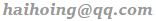OGRE 成功的作品，主要是 天龙八部 和 火炬之光。

## 透视投影和正交投影

``````cam->setProjectionType(Ogre::PT_ORTHOGRAPHIC);
``````

## setDirection

`setDirection` 不支持以 `Ogre::Vector3::UNIT_Y` 为参数。 原因：比如 `setDirection` 为向纸面内看物体 A，此时 Ogre 的显示为 A，而不是倒立的 A，这是因为 `Ogre::Vector3::UNIT_Y` 是 Ogre 默认的 'UP' 视角。 所以 `setDirection(Ogre::Vector3::UNIT_Y)` 会出错，需要先设定向上的坐标轴：

``````cam->setFixedYawAxis(true, gre::Vector3::NEGATIVE_UNIT_Z);
cam->setDirection(0.0f, -1.0f, 0.0f);
``````

## 姿态角（Euler 角）

• Yaw 是围绕当前坐标系的 Y 轴旋转。
• Roll 是围绕当前坐标系的 X 轴旋转。
• Pitch 是围绕当前坐标系的 Z 轴旋转。
``````// 2 的代码写的麻烦一点就是：
cam->setFixedYawAxis(true, Ogre::Vector3::NEGATIVE_UNIT_Z);
cam->setDirection(1.0f, 0.0f, 0.0f);
cam->yaw(Ogre::Degree(90));
// 改变了 UP 之后，当前坐标系也不清晰了，也可以这样写
cam->setFixedYawAxis(true, Ogre::Vector3::NEGATIVE_UNIT_Z);
cam->setDirection(1.0f, 0.0f, 0.0f);
cam->rotate(Ogre::Vector3(0.0f, 0.0f, 1.0f), Ogre::Degree(-90));
// 也可以利用四元数旋转
cam->setFixedYawAxis(true, Ogre::Vector3::NEGATIVE_UNIT_Z);
cam->setDirection(1.0f, 0.0f, 0.0f);
cam->rotate(Ogre::Quaternion(Ogre::Degree(-90), Ogre::Vector3(0.0f, 0.0f, 1.0f)));
``````

## 矩阵的方法

``````世界矩阵 => 摄像矩阵 (ViewMatrix) => 投影矩阵 (ProjectionMatrix)
``````

``````1 0  0 0
0 0 -1 0
0 1  0 -1000 // -1000 是摄像机的位置
0 0  0 1
``````
``````Ogre::Matrix4 mx = Ogre::Matrix4::IDENTITY;
mx = 0.0f;
mx = -1.0f;
mx = 1.0f;
mx = 0.0f;
mx = -1000.f;
cam->setCustomViewMatrix(true, mx);
``````

## 四元数的方法

``````cam->setOrientation(Ogre::Quaternion(Ogre::Degree(-90), Ogre::Vector3(1.0f, 0.0f, 0.0f)));
``````

``````float w = cos(angle / 2);
float x = axis.x * sin(angle / 2);
float y = axis.y * sin(angle / 2);
float z = axis.z * sin(angle / 2);
``````

``````if (1.0f + src.dotProduct(dir) < 0.0001f) {
// 点乘 =-1.0，那么就是方向相反了
src->yaw(Ogre::Degree(180));
} else {
Ogre::Quaternion quat = src.getRotationTo(dir);
src.rotate(quat);
}
``````

## Refs

If you have any questions or feedback, please reach out.